## Transient incompressible flow SUPG/PSPG Algorithm

 SUPG nsteps #(in) deltaT #(rn) rtolv #(rn) [atolv #(rn)] [stopmaxiter #(in)] [alpha #(rn)] [cmflag #(in)] [deltatltf #(in)] [miflag #(in)] [scaleflag #(in) lscale #(in) uscale #(in) dscale #(in)] [lstype #(in)] [smtype #(in)]

Solves the transient incompressible flow using stabilized formulation based on SUPG and PSPG stabilization terms. The stabilization provides stability and accuracy in the solution of advection-dominated problems and permits usage of equal-order interpolation functions for velocity and pressure. Furthermore, stabilized formulation significantly improves convergence rate in iterative solution of large nonlinear systems of equations.

By changing the value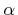, different methods from Generalized mid-point family'' can be chosen, i.e., Forward Euler (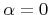), Midpoint rule (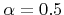), Galerkin (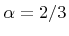), and Backward Euler (). Except the first one, all the methods are implicit and require matrix inversion for solution. Some results form an energy method analysis suggest unconditional stability for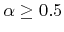for the generalized mid-point family. As far as accuracy is concerned, the midpoint rule is to be generally preferred.

Parameter nsteps determines number of solution steps. Parameter deltaT is time step length used for integration. Alternatively, the load time function can be used to determine time step length for particular solution step. The load time function number is determined by parameter deltatltf and its value evaluated for solution step number should yield the step length.

Parameters rtolv and atolv allow to specify relative and absolute errors norms for residual vector. The equilibrium iteration process will stopped when both error limits are satisfied or when the number of iteration exceeds the value given by parameter stopmaxiter.

If cmflag is given a nonzero value, then consistent mass matrix will be used instead of (default) lumped one.

The algorithm allows to solve the flow of two immiscible fluids in fixed spatial domain (currently only in 2d). This can be also used for solving free surface problems, where one of the fluids should represent air. To enable multi-fluid analysis, user should set parameter miflag. The supported values are described in section 5.5. Please note, that the initial distribution of reference fluid volume should be provided as well as constitutive models for both fluids.

The characteristic equations can be solved in non-dimensional form. To enable this, the scaleflag should have a nonzero value, and the following parameters should be provided: lscale, uscale, and dscale representing typical length, velocity, and density scales.

Parameter lstype allows to select solver for linear system of equations. Parameter smtype allows to select sparse matrix storage scheme. Please note, that the present algorithm leads to a non-symmetrical system matrix. The scheme should be compatible with solver type. See section 5.1 for further details.

Borek Patzak
2018-01-02